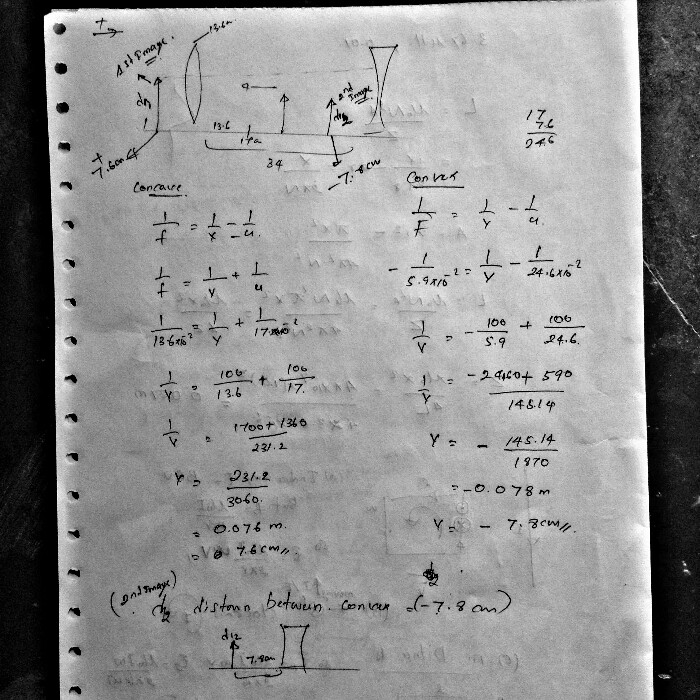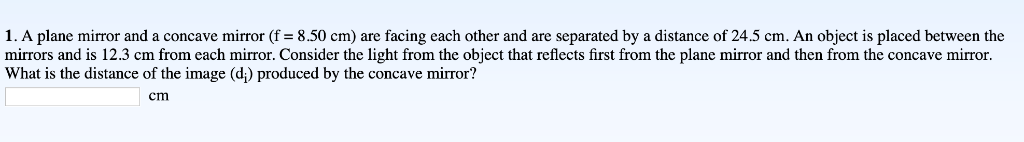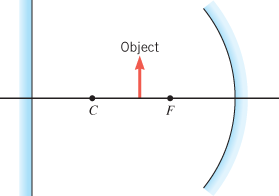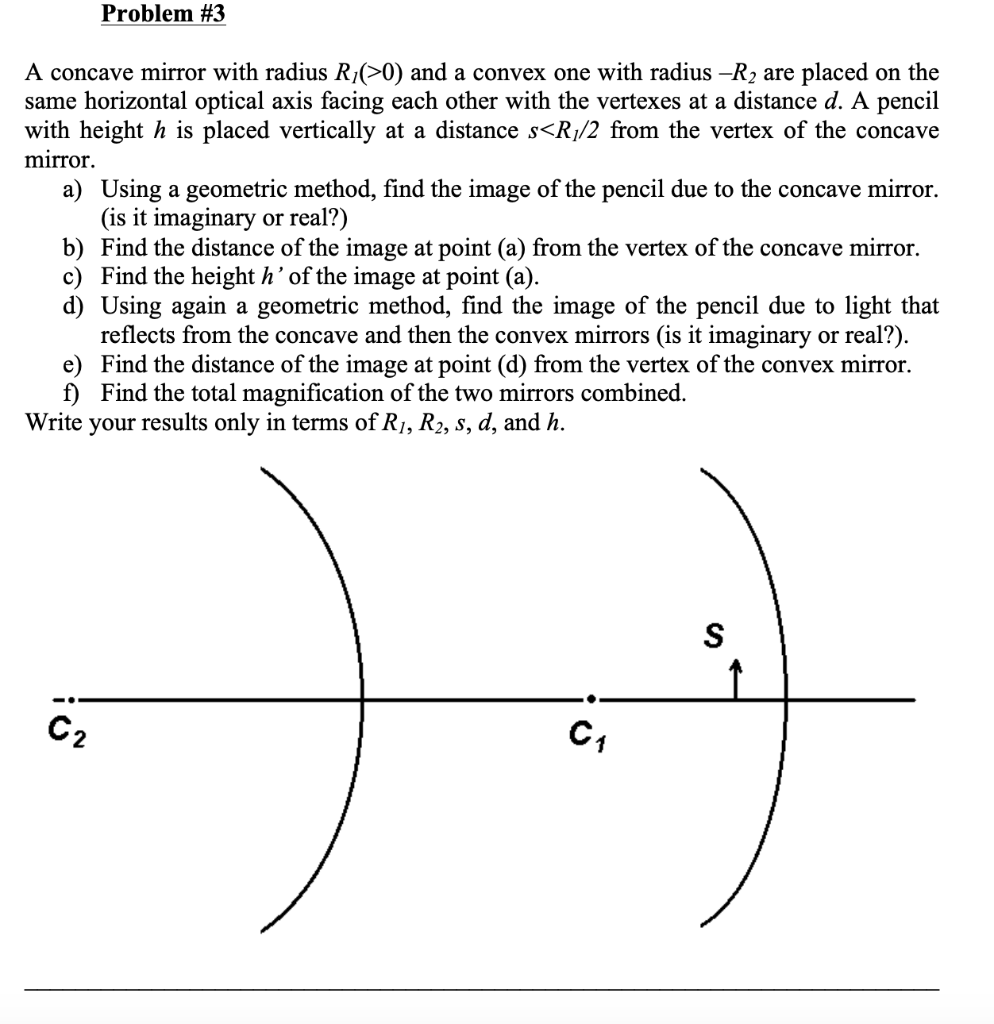Question

# A concave mirror (f1 = 13.6 cm) and a convex mirror (f2 = −5.90 cm) are...

A concave mirror (f1 = 13.6 cm) and a convex mirror (f2 = −5.90 cm) are facing each other and are separated by a distance of 34.0 cm. An object is placed between the mirrors and is 17 cm from each mirror. Consider the light from the object that reflects first from the concave mirror and then from the convex mirror. What is the distance of the image (di2) produced by the convex mirror?.#### Earn Coins

Coins can be redeemed for fabulous gifts.

Similar Homework Help Questions
• ### A concave mirror (f1 = 14.4 cm) and a convex mirror (f2 = ?5.80 cm) are...

A concave mirror (f1 = 14.4 cm) and a convex mirror (f2 = ?5.80 cm) are facing each other and are separated by a distance of 36.0 cm. An object is placed between the mirrors and is 18 cm from each mirror. Consider the light from the object that reflects first from the concave mirror and then from the convex mirror. What is the distance of the image (di2) produced by the convex mirror?.

• ### A concave mirror (f1 = 7.20 cm) and a convex mirror (f2 = ?5.60 cm) are...

A concave mirror (f1 = 7.20 cm) and a convex mirror (f2 = ?5.60 cm) are facing each other and are separated by a distance of 49.6 cm. An object is placed between the mirrors and is 24.8 cm from each mirror. Consider the light from the object that reflects first from the concave mirror and then from the convex mirror. What is the distance of the image (di2) produced by the convex mirror?

• ### A concave mirror (f1 = 13.0 cm) and a convex mirror (f2 = −5.80 cm) are...

A concave mirror (f1 = 13.0 cm) and a convex mirror (f2 = −5.80 cm) are facing each other and are separated by a distance of 35.0 cm. An object is placed between the mirrors and is 17.5 cm from each mirror. Consider the light from the object that reflects first from the concave mirror and then from the convex mirror. What is the distance of the image (di2) produced by the convex mirror?

• ### 2. A convex mirror (f1 = −5.50 cm) and a concave mirror (f2 = 7.80 cm)...

2. A convex mirror (f1 = −5.50 cm) and a concave mirror (f2 = 7.80 cm) are facing each other and are separated by a distance of 16.0 cm. An object is placed between the mirrors and is 8.0 cm from each mirror. Consider the light from the object that reflects first from the convex mirror and then from the concave mirror. What is the distance of the image (di2) produced by the concave mirror? cm

• ### A plane mirror and a concave mirror (f = 8.50 cm) are facing each other and...A plane mirror and a concave mirror (f = 8.50 cm) are facing each other and are separated by a distance of 24.5 cm. An object is placed between the mirrors and is 12.3 cm from each mirror. Consider the light from the object that reflects first from the plane mirror and then from the concave mirror. What is the distance of the image (di) produced by the concave mirror? 1. A plane mirror and a concave mirror (f= 8.50...

• ### A plane mirror and a concave mirror (f = 7.90 cm) are facing each other and...

A plane mirror and a concave mirror (f = 7.90 cm) are facing each other and are separated by a distance of 18.0 cm. An object is placed between the mirrors and is 9.0 cm from each mirror. Consider the light from the object that reflects first from the plane mirror and then from the concave mirror. Draw the ray diagram. What is the distance of the image (di) produced by the concave mirror?

• ### A plane mirror and a concave mirror (f = 6.10 cm) are facing each other and are separated by a distance of 29.0 cm. An object is placed between the mirrors and is 14.5 cm from each mirror. Consider th...A plane mirror and a concave mirror (f = 6.10 cm) are facing each other and are separated by a distance of 29.0 cm. An object is placed between the mirrors and is 14.5 cm from each mirror. Consider the light from the object that reflects first from the plane mirror and then from the concave mirror. Find the location of the image that this light produces in the concave mirror. Specify this distance relative to the concave mirror. Object...

• ### Problem #3 A concave mirror with radius R/(>0) and a convex one with radius -R2 are placed on the...Problem #3 A concave mirror with radius R/(>0) and a convex one with radius -R2 are placed on the same horizontal optical axis facing each other with the vertexes at a distance d. A pencil with height h is placed vertically at a distance s

• ### A concave mirror of 5 cm focal length and a convex mirror of also 5 cm...

A concave mirror of 5 cm focal length and a convex mirror of also 5 cm focal length are 30 cm apart. An object positioned 15 cm from the concave mirror. Let the image first formed by the concave mirror be the object for the convex mirror. Determine the location, orientation, nature (virtual, real), and magnification of the final image formed by the convex mirror.

• ### Concave/Convex mirror

An object 3 cm in size is placed 20 cm in front of aspherical mirros, producing a virual image 40 cm behingthe mirror. Is the mirror concave or convex? Howbig is the image?Is the image upside down or right side up with respect to theobject?